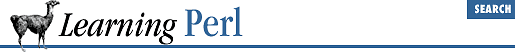home | O'Reilly's CD bookshelfs | FreeBSD | Linux | Cisco | Cisco Exam

### A.3 Chapter 4, Control Structures

1. Here's one way to do it:

```print "What temperature is it? ";
chomp(\$temperature = <STDIN>);
if (\$temperature > 72) {
print "Too hot!\n";
} else {
print "Too cold!\n";
}```

The first line prompts you for the temperature. The second line accepts the temperature for input. The ``` if``` statement on the final 5 lines selects one of two messages to print, depending on the value of ``` \$temperature``` .

2. Here's one way to do it:

```print "What temperature is it? ";
chomp(\$temperature = <STDIN>);
if (\$temperature > 75) {
print "Too hot!\n";
}

elsif (\$temperature < 68) {
print "Too cold!\n";
} else {
print "Just right!\n";
}```

Here, we've modified the program to include a three-way choice. First, the temperature is compared to 75, then to 68. Note that only one of the three choices will be executed each time through the program.

3. Here's one way to do it:

```print "Enter a number (999 to quit): ";
chomp(\$n = <STDIN>);

while (\$n != 999) {
\$sum

+= \$n;
print "Enter another number (999 to quit): ";
chomp(\$n = <STDIN>);
}
print "the sum is \$sum\n";```

The first line prompts for the first number. The second line reads the number from the terminal. The ``` while``` loop continues to execute as long as the number is not 999.

The += operator accumulates the numbers into the ``` \$sum``` variable. Note that the initial value of ``` \$sum``` is ``` undef``` , which makes a nice value for an accumulator, because the first value added in will be effectively added to ``` 0``` (remember that ``` undef``` used as a number is zero).

Within the loop, we must prompt for and receive another number, so that the test at the top of the loop is against a newly entered number.

When the loop is exited, the program prints the accumulated results.

Note that if you enter ``` 999``` right away, the value of ``` \$sum``` is not zero, but an empty string - the value of ``` undef``` when used as a string. If you want to ensure that the program prints zero in this case, you should initialize the value of ``` \$sum``` in the beginning of the program with ``` \$sum = 0``` .

4. Here's one way to do it:

```print "Enter some strings, end with ^D:\n";
@strings = <STDIN>;
while (@strings) {
print

pop @strings;
}```

First, this program asks for the strings. These strings are saved in the array variable ``` @strings``` , one per element.

The control expression of the ``` while``` loop is ``` @strings``` . The control expression is looking for a single value ( true or false ), and is therefore computing the expression in a scalar context. The name of an array (such as ``` @strings``` ) when used in a scalar context is the number of elements currently in the array. As long as the array is not empty, this number is nonzero and therefore true. This is a very common Perl idiom for "do this while the array is nonempty."

The body of the loop prints a value, obtained by ``` pop``` 'ing off the rightmost element of the array. Thus, because that element has been popped, each time through the loop the array is one element shorter.

You may have considered using subscripts for this problem. As we say, there's more than one way to do it. However, you'll rarely see subscripts in true Perl Hackers' programs because there's almost always a better way.

5. Here's a way to do it without a list:

```

for (\$number = 0; \$number <= 32; \$number++) {
\$square = \$number * \$number;

printf "%5g %8g\n", \$number, \$square;
}```

And here's how to do it with a list:

```

foreach \$number (0..32) {
\$square = \$number * \$number;
printf "%5g %8g\n", \$number, \$square;
}```

These solutions both involve loops, using the ``` for``` and ``` foreach``` statements. The body of the loops are identical, because for both solutions, the value of ``` \$number``` proceeds from 0 to 32 on each iteration.

The first solution uses a traditional C-like ``` for``` statement. The three expressions respectively: set ``` \$number``` to 0, test to see if ``` \$number``` is less than or equal to 32, and increment ``` \$number``` on each iteration.

The second solution uses a C-shell-like ``` foreach``` statement. A list of ``` 33``` elements (0 to 32) is created, using the list contructor. The variable ``` \$number``` is then set to each element in turn.A.2 Chapter 3, Arrays and List DataA.4 Chapter 5, Hashes